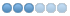# How to implement this cycle (coordinate rotation)

• itsme
• Offline
• Senior Member
•27 Apr 2021 18:54 #207249 by itsme
Hi, i need your helping hand...

I want to achieve the following, but i am not yet familiar with gcodes. I want to mill off the residue of the injection point on moulded parts.
I have a tool setter and a 3d probe on a portal mill.

To achieve this i want to implement the following cycle:

1. Clamp down the parts
2. Measure Probe length with tool setter
3. Measure 3 points around each injection point to determin a plane, 8 points in total
4. Measure tool length with tool setter
5. Mill off excess to match plane for this point, ball end mill with helical path

So how to start? Do i have to program this with a python script or which gcodes can i use.
Are there any sample codes for a problem like this?
Has anyone done something similar and can send me i the right direction?

01 May 2021 20:53 #207570 by andypugh
This sounds like a job that would be much easier with repeatable tool length workholding. It sounds like a manufacturing production process, so is there any budget for that?

Why do you want 8 probed points? 3 should define a plane, and 4 would make for a much simpler routine.

If you probe 4 points in a square then the height at any point inside the square is pretty easy to calculate inside the G-code.

If the area being probed is a higher-order surface then I think that some form of external G-code generator might be needed.
The following user(s) said Thank You: itsme

• itsme
• Offline
• Senior Member
•03 May 2021 07:19 #207706 by itsme
Right now it is just an evaluation example to get into g-coding and lcnc.

Each moulded part has 4 injection points to take care of, so 2 parts make 8 points to flaten. The plane around each point may not be the same.

Probing 4 points (2 for x resp. y) makes sense, if there is no routine for plane rotation xy with 3 points.

Can you give me a hint? Which g-code can be used ot rotate around x, y and z axis?

03 May 2021 14:10 - 03 May 2021 14:12 #207723 by andypugh

Can you give me a hint? Which g-code can be used ot rotate around x, y and z axis?

LinuxCNC has rotation of XY about Z, but there is no arbitrary axis rotation available.

But, a fair amount of calculation can be done in G-code.

We assume a square grid. A more general treatment is possible but the maths becomes more elaborate.

Imagine a capital H.

The working point is somewhere on the bar of the H, it slides up and down.

The proportional distance of the bar from the top corners is calculated to get the Z height of each end of the bar, and then distance of the working point along the bar is calculated. to find the z height of the working point.

We will write a G-code subroutine. This could be passed the probe points parameters, but it is probably clearer to use named global variables. We will use #<_Z1> for "top left Z" etc according to the diagram in the G0code. These would be defined during the probing stage. The _ in a variable name makes it global.

But we will pass X of the working point as #1 and Y as #2 to the subroutine. The answer is returned in <_value>
```G21 G61

; (X1,Y2,Z1)                (X2,Y2,Z2)
;      |                         |
;      |                         |
;      -----------------@---------
;      |                         |
;      |                         |
;      |                         |
;      |                         |
; (X1,Y1,Z4)                (X2,Y1,Z3)

O100 SUB
; normalised positions of the bar and working point
#<pX> = [[#1 - #<_X1>] / [#<_X2> - #<_X1>]]
#<pY> = [[#2 - #<_Y1>] / [#<_Y2> - #<_Y1>]]

; Z height of left and right ends of bar
#<ZL> = [#<_Z4> + #<pY> * [#<_Z1> - #<_Z4>]]
#<ZR> = [#<_Z3> + #<pY> * [#<_Z2> - #<_Z3>]]

(DEBUG, pX = #<pX> pY = #<pY>)

; and return the result
O100 return [#<ZL> + #<pX> * [#<ZR> - #<ZL>]]
O100 endsub

; test data
#<_X1> = -20
#<_X2> = 20
#<_Y1> = -20
#<_Y2> = 20
#<_Z1> = 10
#<_Z2> = -10
#<_Z3> = -15
#<_Z4> = 20

;each corner should return the Z -height of the corner
O100 CALL [-20] 
(DEBUG, #<_value>)
O100 CALL  
(DEBUG, #<_value>)
O100 CALL [-20] [-20]
(DEBUG, #<_value>)
O100 CALL  [-20]
(DEBUG, #<_value>)

;and an arbitrary point
O100 CALL  [-12]
(DEBUG, #<_value>)

; Trace the plane for the backplot
G0 X #<_X1> Y #<_Y1> Z#<_Z4>
G0 X #<_X1> Y #<_Y2> Z#<_Z1>
G0 X #<_X2> Y #<_Y2> Z#<_Z2>
G0 X #<_X2> Y #<_Y1> Z#<_Z3>
G0 X #<_X1> Y #<_Y1> Z#<_Z4>

; And a circle of 4 quadrants in that plane
G1 F1000
O100 call  
G0 X20 Y0 Z#<_value>
O100 call  [-20]
G2 X0 Y-20 Z#<_value> R20
O100 call [-20] 
G2 X-20 Y0 Z#<_value> R20
O100 call  
G2 X0 Y20 Z#<_value> R20
O100 call  
G2 X20 Y0 Z#<_value> R20

M2```

In practice you would probably want to be using rather smaller segments to follow the probed surface better.
Last edit: 03 May 2021 14:12 by andypugh.
The following user(s) said Thank You: itsme

•